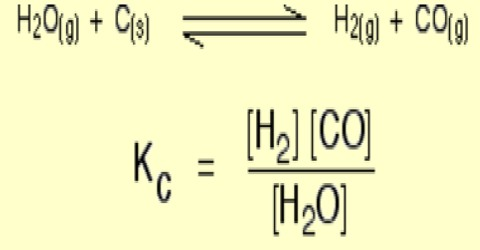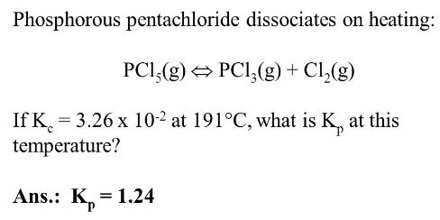Chemistry

# Homogeneous EquilibriaHomogeneous Equilibria

A homogeneous equilibrium has the whole thing present in the similar phase. The common examples contain reactions where everything is a gas, or everything is present in the similar solution. Examples of few homogeneous equilibrium systems are given below:

(i) Dissociation of nitrogen tetroxide: The dissociation of nitrogen tetroxide is represented by the equation

N2O4 (g) ↔ 2NO2

for which the equilibrium constant is,

KP = [(PNO2)2 / PN2O4]

PNO2 and PN2O4 being the partial pressure of NO2 and N2O4 respectively.

The experimental method applied in determining the equilibrium constant consists in measuring the total pressure at equilibrium in a flask of known volume in which a known mass of N2O4 was placed. Using the ideal gas equation, the apparent molecular mass

of the partially dissociated gas is calculated and equation is used to determine the degree of dissociation at equilibrium. If a represents the degree of dissociation, (1- α) is proportional to the number of moles of undissociated N2O4 and 2α is proportional to the number of moles of NO2 so that (1- α + 2α) or (1 + a) is proportional to the total number of moles.

If P is the total pressure, the partial pressures are given by

PNO2 = {2α / (1 + α)}P and PN2O4 = {2α / (1 + α)}P

Hence, KP = [{2α / (1 + α)}P]2 / {2α / (1 + α)}P

= {4α2 / (1 – α2)}P

(2) Dissociation of phosphorus pentachloride:When heated, phosphorus pentachloride dissociates and the reaction is represented by the following equation:

PCl5 (g) ↔ PCl3 (g) + Cl2 (g)

The equilibrium constant for this reaction is,

KC = [PCl3] [Cl2] / [PC15]

which can he determined by measuring the degree of dissociation al the given temperature as in the case of the dissociation of nitrogen tetroxide.

(3) Formation and decomposition of hydrogen iodide gas:

A classical example of gaseous homogeneous equilibrium is the following reaction above 4000C.

2 HI (g) ↔ H2 (g) + I2 (g)

This reaction was extensively studied by Bodenstein and others. Known quantities of hydrogen iodide were sealed in quartz vessels of known volume and heated at the desired temperature for sufficient time until equilibrium was reached. The vessels were then suddenly cooled and the contents analyzed for iodine by titration with standard sodium thiosulphate solution. As the equation above shows, the concentration of hydrogen is the same as that of iodine while the concentration of hydrogen iodide at equilibrium is the initial concentration of hydrogen iodide minus twice the concentration of iodine.